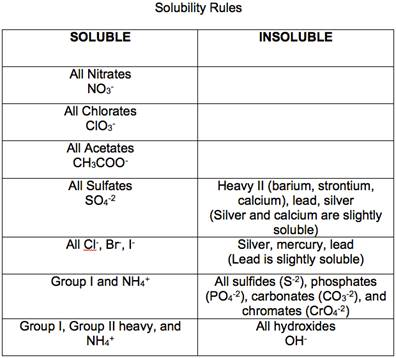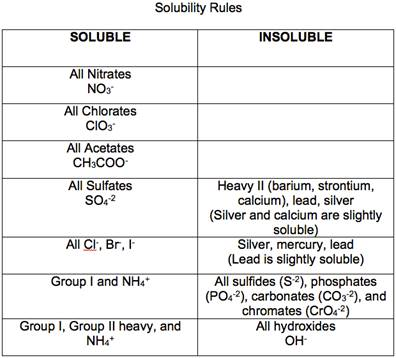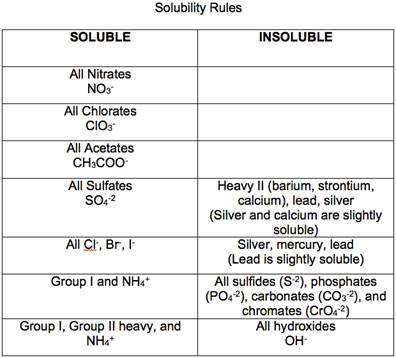# Using the solubility guidelines (Figure 3.10), predict whether each of the following is insoluble or soluble in water. (a) (NH 4 ) 2 CO 3 (b) ZnSO 4 (c) NiS (d) BaSO 4### Chemistry & Chemical Reactivity

10th Edition
John C. Kotz + 3 others
Publisher: Cengage Learning
ISBN: 9781337399074### Chemistry & Chemical Reactivity

10th Edition
John C. Kotz + 3 others
Publisher: Cengage Learning
ISBN: 9781337399074

#### Solutions

Chapter
Section
Chapter 17, Problem 39PS
Textbook Problem

## Using the solubility guidelines (Figure 3.10), predict whether each of the following is insoluble or soluble in water.(a) (NH4)2CO3(b) ZnSO4(c) NiS(d) BaSO4

Expert Solution

a)

Interpretation Introduction

Interpretation:

The following ion (NH4)2CO3 is insoluble or soluble in water by using the solubility gude lines has to be determined.

Concept introduction:

Precipitation reactions are exchange reactions in which one of the products is a water - insoluble compounds.

For example -CaCO3

CaCl2(aq) + Na2CO3(aq)  CaCO3(s) + 2NaCl(aq)

The solubility of a compound is defined as the amount of that substance that can be dissolved in a given quantity of a solvent.

All ionic compounds of alkali metals and ammonium ions are soluble in water.### Explanation of Solution

(NH4)2

Expert Solution

b)

Interpretation Introduction

Interpretation:

The following ion ZnSO4 is insoluble or soluble in water by using the solubility gude lines has to be determined.

Concept introduction:

Precipitation reactions are exchange reactions in which one of the products is a water - insoluble compounds.

For example -CaCO3

CaCl2(aq) + Na2CO3(aq)  CaCO3(s) + 2NaCl(aq)

The solubility of a compound is defined as the amount of that substance that can be dissolved in a given quantity of a solvent.

All ionic compounds of alkali metals and ammonium ions are soluble in water.Expert Solution

c)

Interpretation Introduction

Interpretation:

The following ion NiS is insoluble or soluble in water by using the solubility gude lines has to be determined.

Concept introduction:

Precipitation reactions are exchange reactions in which one of the products is a water - insoluble compounds.

For example -CaCO3

CaCl2(aq) + Na2CO3(aq)  CaCO3(s) + 2NaCl(aq)

The solubility of a compound is defined as the amount of that substance that can be dissolved in a given quantity of a solvent.

All ionic compounds of alkali metals and ammonium ions are soluble in water.Expert Solution

d)

Interpretation Introduction

Interpretation:

The following ion BaSO4 is insoluble or soluble in water by using the solubility gude lines has to be determined.

Concept introduction:

Precipitation reactions are exchange reactions in which one of the products is a water - insoluble compounds.

For example -CaCO3

CaCl2(aq) + Na2CO3(aq)  CaCO3(s) + 2NaCl(aq)

The solubility of a compound is defined as the amount of that substance that can be dissolved in a given quantity of a solvent.

All ionic compounds of alkali metals and ammonium ions are soluble in water.### Want to see the full answer?

Check out a sample textbook solution.See solution

### Want to see this answer and more?

Bartleby provides explanations to thousands of textbook problems written by our experts, many with advanced degrees!

See solution